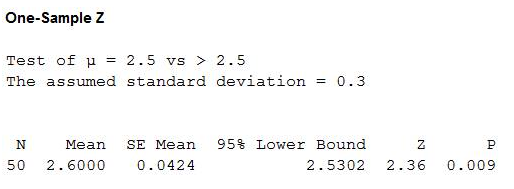# The article “Supply Voltage Quality in Low-Voltage Industrial Networks of Estonia” (T. Vinnal, K. Janson, et al., Estonian Journal of Engineering, 2012:102–126) presents voltage measurements for a sample of 66 industrial networks in Estonia. Assume the rated voltage for these networks is 232 V. The sample mean voltage was 231.7 V with a standard deviation of 2.19 V. Let μ represent the population mean voltage for these networks. a) Find the P-value for testing H0: μ = 232 versus H1: μ ≠ 232. b) Either the mean voltage is not equal to 232, or the sample is in the most extreme _____% of its distribution.

Question
37 views

The article “Supply Voltage Quality in Low-Voltage Industrial Networks of Estonia” (T. Vinnal, K. Janson, et al., Estonian Journal of Engineering, 2012:102–126) presents voltage measurements for a sample of 66 industrial networks in Estonia. Assume the rated voltage for these networks is 232 V. The sample mean voltage was 231.7 V with a standard deviation of 2.19 V. Let μ represent the population mean voltage for these networks. a) Find the P-value for testing H0: μ = 232 versus H1: μ ≠ 232. b) Either the mean voltage is not equal to 232, or the sample is in the most extreme _____% of its distribution.

check_circle

Step 1

Note: here, MINITAB software is used to obtain the hypothesis test for proportion.

a.

In a sample of 50 batches, the mean pH was 2.6 with a standard deviation of 0.3.

Tests statistic:

Software Procedure:

Step-by-step procedure to obtain the test statistic using the MINITAB software:

• Choose Stat > Basic Statistics > 1-Sample Z.
• In Summarized data, enter the sample size as 50 and mean as 2.6.
• In Standard deviation, enter a value for s as 0.3.
• In Perform hypothesis test, enter the hypothesized mean as 2.5.
• Check Options; enter Confidence level as 95%.
• Choose greater than in alternative.
• Click OK.

Output using the MINITAB software is given below:Step 2

From the MINITAB output, the test statistic is 2.36.

P-value:

Software Procedure:

Step-by-step procedure to obtain the P- value using the MINITAB software:

• Choose Graph > Probability Distribution Plot choose View Probability > OK.
• From Distribution, choose ‘Normal’ distribution.
• Click the Shaded Area tab.
• Choose X Value and Right Tail for the region of the curve to shade.
• Enter the data value as 2.36.
• Click OK.

Output using the MINIT...

### Want to see the full answer?

See Solution

#### Want to see this answer and more?

Solutions are written by subject experts who are available 24/7. Questions are typically answered within 1 hour.*

See Solution
*Response times may vary by subject and question.
Tagged in